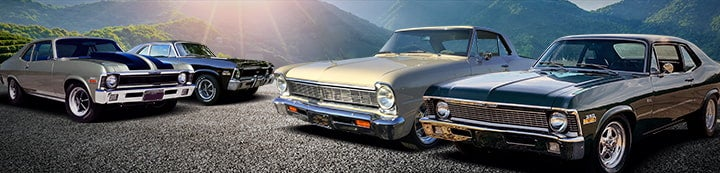41 - 45 of 45 Posts

#### stock z/28

·
##### Registered
Joined
·
3,416 Posts
I wonder what the load actually is on the bolts, figuring the weight and length on a typical iron head small block and the bolt spread on the bellhousing?

It would be interesting to me.

#### Paul Wright

·
Joined
·
8,405 Posts
Coincidently, we are studying this in Physics. I'm pretty sure the load difference between the top and bottom bolts is a Vector problem. Might also require calculating center of mass.
I think the spacers help resist bending forces and the bolts just provide clamping force. The weight applied plus the bolt torque has to be considered when calculating total strain.
While the load may not be super high, I wouldn't chance a \$7,000 engine with grade 2 bolts, especially with all the poor quality, Chinese bolts at hardware stores.
It would be interesting to see what the actual loads would be. I'll have to find the equations and submit a report.

#### Mike Goble

·
##### Registered
Joined
·
9,964 Posts
Here's an introductory course on the importance of stress analysis:

http://www.gendertree.com/a_stress_analysis_of_a_strapless.htm

A rough calculation on the stress put on the bolts would be a ratio of the distance of the engine's c/g from the mounting flange to the distance between the bolts. Let's say the c/g of the motor is 16" from the flange and the bolts are 8" apart. If it's 2/1, the stress on the upper bolts is about double the weight of the engine.
The same rough calculation would apply to the estimate of the stress on the mounting head, the ratio of the diameter of the tube to the distance from the end of the tube to the c/g of the motor. If the tube is 2.5" in diameter and the c/g is 20" from the weld, it's about an 8:1 ratio.

It's interesting that so much time is spent gnashing teeth over the rating of the engine mounting bolts, yet so little time is spent calculating the forces on the cheap Chinese engine hoist and the low-priced chain that comes with it.

#### The Big Al

·
Joined
·
15,966 Posts
Now y'all done it!

After 35 years of using any bolt I could find that would fit.
The engine will now fall off and crash to the floor because now I know the bolts are not rated for the stress of holding up the engine.

They worked perfect until someone pointed this out.·